# Test: Differentiation Implicit Functions

## 10 Questions MCQ Test Mathematics (Maths) Class 12 | Test: Differentiation Implicit Functions

Description
This mock test of Test: Differentiation Implicit Functions for JEE helps you for every JEE entrance exam. This contains 10 Multiple Choice Questions for JEE Test: Differentiation Implicit Functions (mcq) to study with solutions a complete question bank. The solved questions answers in this Test: Differentiation Implicit Functions quiz give you a good mix of easy questions and tough questions. JEE students definitely take this Test: Differentiation Implicit Functions exercise for a better result in the exam. You can find other Test: Differentiation Implicit Functions extra questions, long questions & short questions for JEE on EduRev as well by searching above.
QUESTION: 1

Solution:
QUESTION: 2

### Differentiate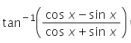with respect to x.

Solution:

(cosx − sinx)/(cosx + sinx) = (1 − tanx)/(1 + tanx)
tan(A − B) = (tanA − tanB)/(1 + tanAtanB)
= tan(π/4−x)
putting this value in question.
tan−1 tan(π/4−x)
π/4 − x.

so d(π/4 − x)/dx = -1

QUESTION: 3

### The dervative id sec-1 x is

Solution:

y = sec−1 x
by rewriting in terms of secant,
⇒ secy = x
by differentiating with respect to x,
⇒ secy tany y'= 1 by dividing by secy tany,
⇒ y'= 1/secy tany
since secy = x and tanx = √sec2y − 1 = √x2−1
⇒ y'= 1/x√x2−1
Hence, d/dx(sec−1x) = 1/x√x2−1

QUESTION: 4

If y + sin y = 5x, then the value of dy/dx is

Solution:

y + sin y = 5x
dy/dx + cos ydy/dx = 5
dy/dx = 5/(1+cos y)

QUESTION: 5

Derivative of tan-1 x is

Solution:
QUESTION: 6

If xy = 2, then dy/dx  is

Solution:

xy = 2
x dy/dx + y = 0
x dy/dx = -y
dy/dx = -y/x

QUESTION: 7

If 3 sin(xy) + 4 cos (xy) = 5, then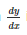= .....

Solution:

3sinxy + 4cosxy = 5
⇒ 5(3/5 sinxy + 4/5 cosxy) = 5
⇒ (3/5 sinxy + 4/5 cosxy) = 1
now (3/5)²+(4/5)² = 1
so let, 3/5 =   cosA
⇒ 4/5 = sinA
So , (3/5 sinxy + 4/5 cosxy) = 1
⇒ (cosAsinxy + sinAcosxy) = 1
⇒ sin(A+xy) = 1
⇒ A + xy = 2πk + π/2 (k is any integer)
⇒ sin⁻¹(4/5) + xy = 2πk + π/2
differenciating both sides with respect to x
0 + xdy/dx + y = 0
dy/dx = -y/x

QUESTION: 8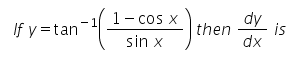Solution:

y = tan-1(1-cosx)/sinx
y = tan-1{2sin2(x/2)/(2sin(x/2)cos(x/2)}
y = tan-1{tan x/2}
y = x/2  => dy/dx = 1/2

QUESTION: 9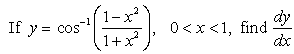Solution:

cos-1[(1-x2)/(1+x2)]
Put x = tanθ
y = cos-1(1 - tan2θ)/(1 + tan2θ)
y = cos-1(cos2θ)
y = 2θ……………….(1)
Put θ = tan-1 x in eq(1)
y = 2(tan-1 x)
dy/dx = 2(1/(1+x2))
dy/dx = 2/(1+x2)

QUESTION: 10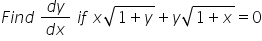Solution: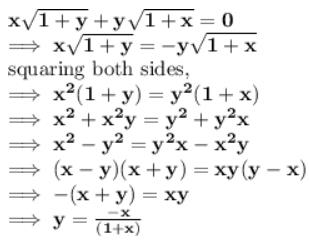now differentiate y with respect to x,
dy/dx = -{[x d/dx(1+x) - (1+x)dx/dx]}/(1+x2)
= -1/(1+x)2